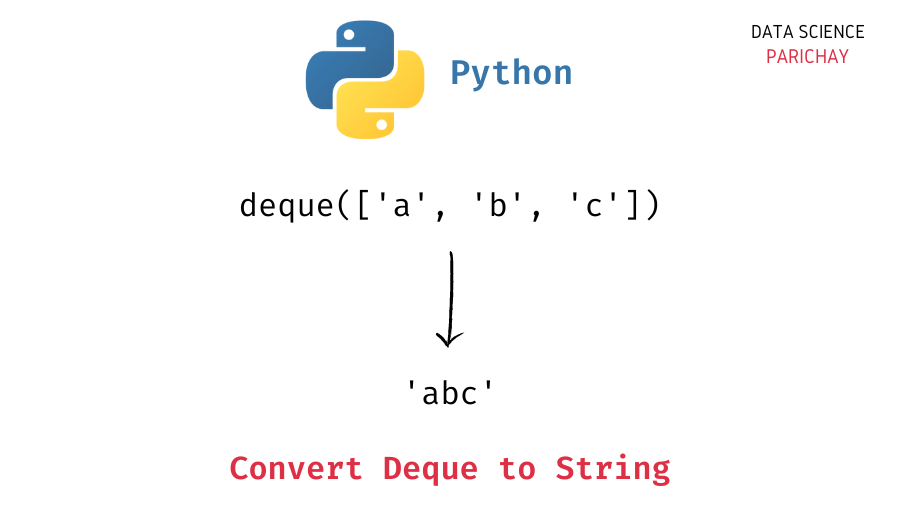# Python – Convert a deque of strings to a single string

In this tutorial, we will look at how to convert a deque object containing string values to a string in Python with the help of some examples.

## `deque` in Python

The `collections` module in Python comes with a `deque` class that you can use to implement a stack or queue data structure in Python. Deques support thread-safe, memory efficient appends and pops from either side of the deque with approximately the same O(1) performance in either direction.

In simple terms, you can think of a `deque` in Python as a list-like data structure but with efficient insertion and removal of items from either end (left and right). This makes them very useful when implementing a stack or queue functionality in Python.

## How to convert a deque to a string?

You can use the string `join()` function to convert a deque with only string values to a single string in Python. The idea is to join the string values in the deque together using an empty string with the `join()` function.

The following is the syntax –

```# convert deque of strings to a string
"".join(queue)```

Note that the above code will raise a `TypeError` if any of the values in the deque is not of string type.

## Examples

Let’s now look at some examples of using the above syntax

### Example 1 – Convert a deque of strings to a single string

First, let’s create a deque with some string elements.

📚 Data Science Programs By Skill Level

Introductory

Intermediate ⭐⭐⭐

🔎 Find Data Science Programs 👨‍💻 111,889 already enrolled

Disclaimer: Data Science Parichay is reader supported. When you purchase a course through a link on this site, we may earn a small commission at no additional cost to you. Earned commissions help support this website and its team of writers.

```from collections import deque

# create a deque
queue = deque(["a", "b", "c"])
# print the deque
print(queue)```

Output:

`deque(['a', 'b', 'c'])`

Here, we imported the `deque` class from the `collections` module and created a deque `queue` with some string values.

Let’s now convert the above deque to a single-string value.

```# convert deque to string
"".join(queue)```

Output:

`'abc'`

Here, we used the string `join()` function to concatenate the values in the deque `queue` with an empty string.

### Example 2 – Convert a deque of numbers to a string

If you apply the string `join()` on a deque containing non-string values, you’ll get a `TypeError`.

```# create a deque
queue = deque([1, 2, 3])
# convert deque to string
"".join(queue)```

Output:

```---------------------------------------------------------------------------
TypeError                                 Traceback (most recent call last)
Input In , in <module>
2 queue = deque([1, 2, 3])
3 # convert deque to string
----> 4 "".join(queue)

TypeError: sequence item 0: expected str instance, int found```

To convert a deque of numbers to a single string, you can use a list comprehension to first get values in the deque as strings in a list and then concat them together using the string `join()` function.

```# convert deque to string
"".join([str(val) for val in queue])```

Output:

`'123'`

We get the values in the deque as a single string.

You might also be interested in –

•